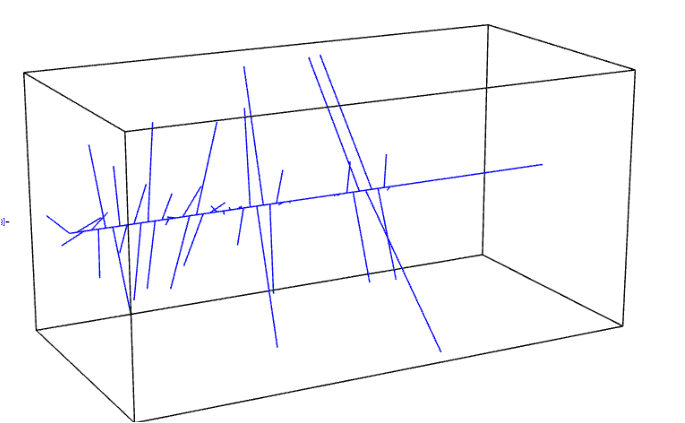# Mathematica: DiscretePlot type plot with x values from a list

• Mathematica
• Swamp Thing

#### Swamp Thing

TL;DR Summary
Is it possible to get something that looks like DiscretePlot, but taking both the X *and* Y values from a list?
I would like vertical lines with dots at the top of them, with the x-positions and heights coming from
{{1.2, 3.5}, {2.3, 4.2}, {5.5, 6.7}} for example.

Try ListPlot with the option Filling->Axis

•Swamp Thing and DrClaude
That's exactly what I needed, thanks.

And another question, if I may: I have used ListPointPlot3D to show some complex numbers as a function of some ##t## that goes along the length of the box:However, the Filling->Axis doesn't help here because it sends each line downwards rather than towards the ##t## axis.

I had to doctor the data and insert ##0 + i0## before and after each complex number to get the above plot -- so ListPointPlot3D is probably the wrong type of plot for this.

What is the best way to do this?

Last edited:

## 1. How do I create a DiscretePlot type plot in Mathematica with x values from a list?

To create a DiscretePlot type plot in Mathematica with x values from a list, you can use the syntax DiscretePlot[data, {x, list}]. This will plot the data points at the x values specified in the list.

## 2. Can I use a different marker style for each data point in a DiscretePlot?

Yes, you can use the PlotMarkers option in the DiscretePlot function to specify a different marker style for each data point. For example, DiscretePlot[data, {x, list}, PlotMarkers -> {"●", "▲", "■"}] will use a circle, triangle, and square marker for the first, second, and third data points respectively.

## 3. How do I change the size and color of the markers in a DiscretePlot?

You can use the PlotStyle option in the DiscretePlot function to specify the size and color of the markers. For example, DiscretePlot[data, {x, list}, PlotStyle -> Directive[PointSize[0.02], Red]] will use red markers with a size of 0.02.

## 4. Can I add labels to the x-axis and y-axis in a DiscretePlot?

Yes, you can use the FrameLabel option in the DiscretePlot function to add labels to the x-axis and y-axis. For example, DiscretePlot[data, {x, list}, FrameLabel -> {"x-axis", "y-axis"}] will add labels to the axes.

## 5. Is it possible to add a legend to a DiscretePlot?

Yes, you can use the PlotLegends option in the DiscretePlot function to add a legend. For example, DiscretePlot[data, {x, list}, PlotLegends -> {"Data"}] will add a legend with the label "Data". You can also specify the position of the legend using the LegendPosition option.